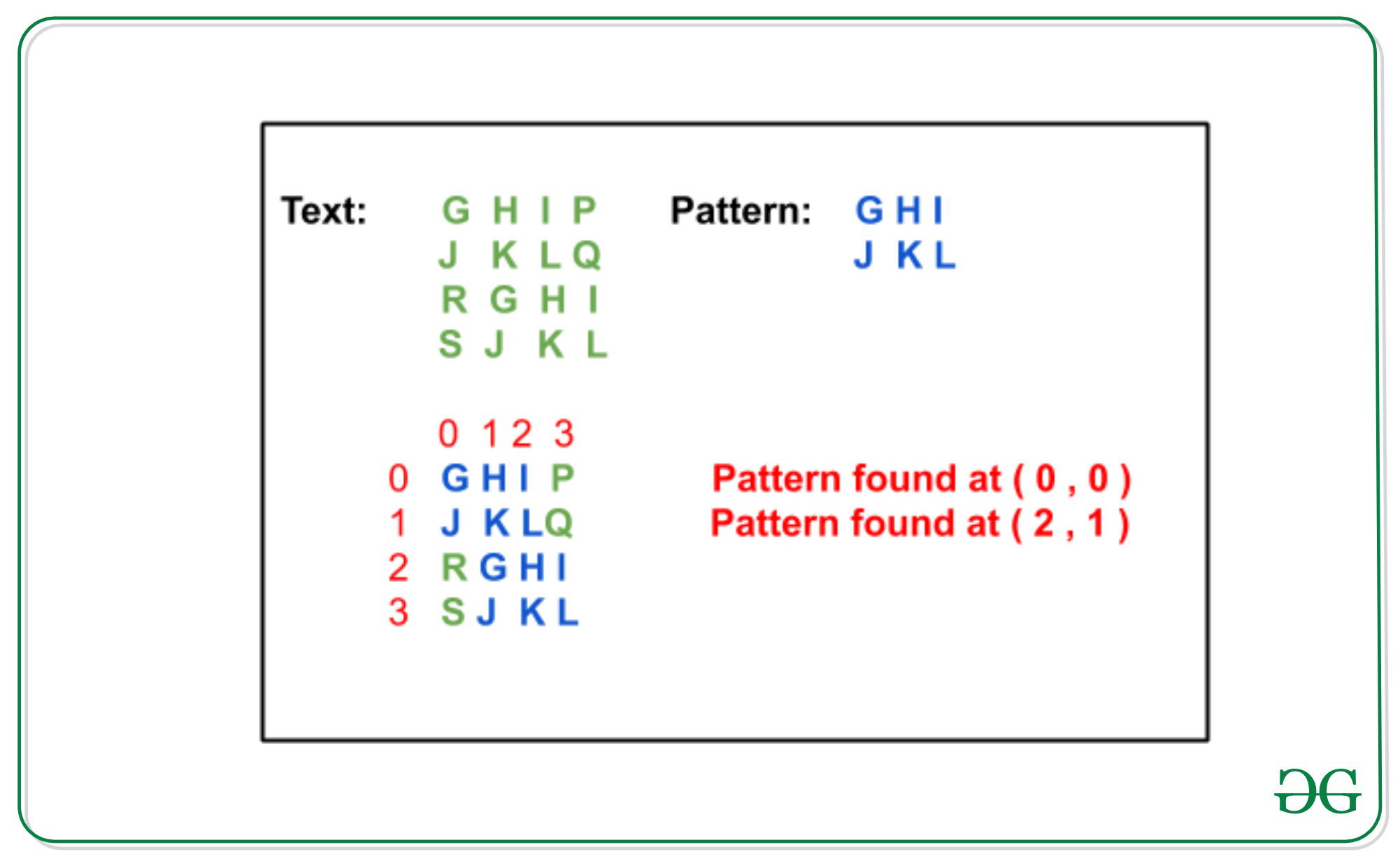# Rabin-Karp algorithm for Pattern Searching in Matrix

Given matrices txt[][] of dimensions m1 x m2 and pattern pat[][] of dimensions n1 x n2, the task is to check whether a pattern exists in the matrix or not and if yes then print the top mot indices od the pat[][] in txt[][]. It is assumed that m1, m2 ≥ n1, n2

Examples:

```Input:
txt[][] = {{G, H, I, P}
{J, K, L, Q}
{R, G, H, I}
{S, J, K, L}
}
pat[][] = {{G, H, I},
{J, K, L}
}
Output:
Pattern found at ( 0, 0 )
Pattern found at ( 2, 1 )
Explanation:Input:
txt[][] = { {A, B, C},
{D, E, F},
{G, H, I}
}
pat[][] = { {E, F},
{H, I}
}
Output:
Pattern found at (1, 1)
```

Approach: In order to find a pattern in a 2-D array using Rabin-Karp algorithm, consider an input matrix txt[m1][m2] and a pattern pat[n1][n2]. The idea is to find the hash of each columns of mat[][] and pat[][] and compare the hash values. For any column if hash values are equals than check for the corresponding rows values. Below are the steps:

1. Find the hash values of each column for the first N1 rows in both txt[][] and pat[][] matrix.
2. Apply Rabin-Karp Algorithm by finding hash values for the column hashes found in step 1.
3. If a match is found compare txt[][] and pat[][] matrices for the specific rows and columns.
4. Else slide down the column hashes by 1 row in the txt matrix using a rolling hash.
5. Repeat steps 2 to 4 for all the hash values and if we found any pat[][] match in txt[][] then print the indices of top most cell in the txt[][].

To find the hash value: In order to find the hash value of a substring of size N in a text using rolling hash follow below steps:

1. Remove the first character from the string: hash(txt[s:s+n-1])-(radix**(n-1)*txt[s])%prime_number

Below is the implementation of the above approach:

## Python3

 `# Python implementation for the  ` `# pattern matching in 2-D matrix ` ` `  `# Function to find the hash-value  ` `# of the given columns of text ` `def` `findHash(arr, col, row): ` `    ``hashCol ``=` `[] ` `    ``add ``=` `0` `    ``radix ``=` `256` ` `  `    ``# For each column ` `    ``for` `i ``in` `range``(``0``, col): ` ` `  `        ``for` `j ``in` `reversed``(``range``(``0``, row)): ` `            ``add ``=` `add ``+` `(radix``*``*``(row``-``j``-``1``) ``*`  `                         ``ord``(arr[j][i]))``%` `101` `        ``hashCol.append(add ``%` `101``); ` `        ``add ``=` `0` `    ``return` `hashCol ` ` `  `# Function to check equality of the  ` `# two strings ` `def` `checkEquality(txt, row, col, flag): ` `    ``txt ``=` `[txt[i][col:patCol ``+` `col]  ` `           ``for` `i ``in` `range``(row, patRow ``+` `row)] ` ` `  `# If pattern found ` `    ``if` `txt ``=``=` `pat: ` `        ``flag ``=` `1` `        ``print``(``"Pattern found at"``, \ ` `              ``"("``, row, ``", "``, col, ``")"``) ` `    ``return` `flag ` `     `  `# Function to find the hash value of ` `# of the next column using rolling-hash ` `# of the Rabin-karp ` `def` `colRollingHash(txtHash, nxtRow): ` ` `  `    ``radix ``=` `256` ` `  `    ``# Find the hash of the matrix ` `    ``for` `j ``in` `range``(``len``(txtHash)): ` `        ``txtHash[j] ``=` `(txtHash[j]``*``radix \ ` `                      ``+` `ord``(txt[nxtRow][j]))``%` `101` `        ``txtHash[j] ``=` `txtHash[j] ``-` `(radix``*``*``(patRow) ``*`  `                     ``ord``(txt[nxtRow``-``patRow][j]))``%` `101`  `        ``txtHash[j] ``=` `txtHash[j]``%` `101` `    ``return` `txtHash ` `     `  ` `  `# Function to match a pattern in  ` `# the given 2D Matrix ` `def` `search(txt, pat): ` `     `  `# List of the hashed value for ` `    ``# the text and pattern columns ` `    ``patHash ``=` `[] ` `    ``txtHash ``=` `[] ` ` `  `    ``# Hash value of the  ` `    ``# pat_hash and txt_hash ` `    ``patVal ``=` `0` `    ``txtVal ``=` `0`  ` `  `    ``# Radix value for the input characters ` `    ``radix ``=` `256` `     `  `    ``# Variable to determine if ` `    ``# pattern was found or not ` `    ``flag ``=` `0` `     `  `    ``# Function call to find the ` `    ``# hash value of columns ` `    ``txtHash ``=` `findHash(txt, txtCol, patRow)   ` `    ``patHash ``=` `findHash(pat, patCol, patRow) ` `     `  `    ``# Calculate hash value for patHash ` `    ``for` `i ``in` `range``(``len``(patHash)): ` `        ``patVal ``=` `patVal \ ` `                 ``+` `(radix``*``*``(``len``(patHash)``-``i``-``1``) ``*`  `                 ``patHash[i]``%` `101``) ` `    ``patVal ``=` `patVal ``%` `101` `     `  `     `  `    ``# Applying Rabin-Karp to compare ` `    ``# txtHash and patHash ` `    ``for` `i ``in` `range``(patRow``-``1``, txtRow): ` `        ``col ``=` `0` `        ``txtVal ``=` `0` `         `  `        ``# Find the hash value txtHash  ` `        ``for` `j ``in` `range``(``len``(patHash)): ` `            ``txtVal ``=` `txtVal\ ` `                     ``+` `(radix``*``*``(``len``(patHash)``-``j``-``1``) ``*`  `                     ``txtHash[j])``%` `101` `        ``txtVal ``=` `txtVal ``%` `101` `         `  `        ``if` `txtVal ``=``=` `patVal: ` `            ``flag ``=` `checkEquality(\ ` `                     ``txt, i ``+` `1``-``patRow, col, flag) ` `             `  `        ``else``: ` ` `  `            ``# Roll the txt window by one character  ` `            ``for` `k ``in` `range``(``len``(patHash), ``len``(txtHash)): ` ` `  `                ``txtVal ``=` `txtVal \ ` `                         ``*` `radix ``+` `(txtHash[k])``%` `101` `                ``txtVal ``=` `txtVal \ ` `                         ``-` `(radix``*``*``(``len``(patHash)) ``*` `                         ``(txtHash[k``-``len``(patHash)]))``%` `101` `                ``txtVal ``=` `txtVal ``%` `101` `                ``col ``=` `col ``+` `1` ` `  `                ``# Check if txtVal and patVal are equal ` `                ``if` `patVal ``=``=` `txtVal: ` `                    ``flag ``=` `checkEquality(\ ` `                           ``txt, i ``+` `1``-``patRow, col, flag)    ` `                ``else``: ` `                    ``continue` `                 `  `        ``# To make sure i does not exceed txtRow ` `        ``if` `i ``+` `1``

Output:

```Pattern found at ( 1 ,  1 )
```
DSA Self Paced Course at a student-friendly price and become industry ready.

My Personal Notes arrow_drop_upCheck out this Author's contributed articles.

If you like GeeksforGeeks and would like to contribute, you can also write an article using contribute.geeksforgeeks.org or mail your article to contribute@geeksforgeeks.org. See your article appearing on the GeeksforGeeks main page and help other Geeks.

Please Improve this article if you find anything incorrect by clicking on the "Improve Article" button below.